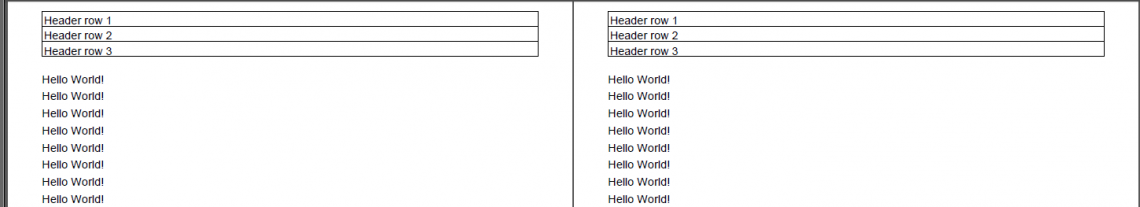Legacy notice!

iText 5 is the previous major version of iText’s leading PDF SDK. iText 5 is EOL, and is no longer developed, although we still provide support and security fixes. Switch your project to iText 8, our latest version which supports the latest PDF standards and technologies.
Check related iText 8 content!

I am using page events to create a header that consists of a table. This table is added to each page in my document, but unfortunately, it overlaps with the rest of my content. How can I avoid this?

Question inspired by the posts on StackOverflow dated February 17, 2016 by Abhimanyu Katoch and Herin

You create your document like this:

``````Document document = new Document();
``````

This means that the lower-left coordinate of your document is `(0, 0)` and the upper-right coordinate is `(595, 842)`.

Then you define margins for the document:

``````document.SetMargins(30, 30, 45, 30);
``````

This means that:

• `Document.Left` = 0 + 30 = 30

• `Document.Right` = 595 - 30 = 565

• `Document.Top` = 842 - 845 = 797

• `Document.Bottom` = 0 + 30 = 30

So when you have this line:

``````table.WriteSelectedRows(0, -1, document.LeftMargin, document.Top, writer.DirectContent);
``````

You actually have:

``````table.WriteSelectedRows(0, -1, 30, 797, writer.DirectContent);
``````

This is wrong because that makes your table overlap with whatever content you are adding inside the margins.

To solve this, you need to calculate the height of the table. See for instance the answer to the question How to define the page size based on the content?

``````table.LockedWidth = true;
Float h = table.TotalHeight;
``````

Now you can use `h` to define the top margin:

``````document.SetMargins(30, 30, 20 + h, 30);
``````

And you can use `Document.Top` to define the `y` position of the table:

``````table.WriteSelectedRows(0, -1,
document.LeftMargin,
document.Top + h + 10,
writer.DirectContent);
``````

With this code, the table will be added inside the margin, leaving 10 user units of white space under the top of the page and 10 user units of white space above the top margin.

Abhimanyu Katoch didn't understand this answer, so I created an example:

In this example, I create a document with some "Hello World" content:

``````public void createPdf(String filename) throws IOException, DocumentException {
// step 1
Document document = new Document(PageSize.A4, 36, 36, 20 + event.getTableHeight(), 36);
// step 2
PdfWriter writer = PdfWriter.getInstance(document, new FileOutputStream(filename));
writer.setPageEvent(event);
// step 3
document.open();
// step 4
for (int i = 0; i ``````

As you can see, I also define a `TableHeader` event. I use this `event` as a page event, but I also need this event when I define the `Document`. I use the following value as top margin:

``````20 + event.getTableHeight()
``````

What does this mean? Let's take a look at the implementation of this event:

``````public class HeaderTable extends PdfPageEventHelper {
protected PdfPTable table;
protected float tableHeight;
table = new PdfPTable(1);
table.setTotalWidth(523);
table.setLockedWidth(true);
tableHeight = table.getTotalHeight();
}

public float getTableHeight() {
return tableHeight;
}

public void onEndPage(PdfWriter writer, Document document) {
table.writeSelectedRows(0, -1,
document.left(),
document.top() + ((document.topMargin() + tableHeight) / 2),
writer.getDirectContent());
}
}
``````

When I create the event, a `PdfPTable` is constructed. I store this `table` as a member variable, along with the height of this table: `tableHeight`.

I use this `tableHeight` when I define the top margin, so that I am 100% sure that the table will fit the margin. I add an additional 20 user units because I don't want the table to stick to the top border of the page:

``````20 + event.getTableHeight()
``````

When I add the table in the `onEndPage()` method, I use the following coordinates:

``````x = document.left()
y = document.top() + ((document.topMargin() + tableHeight) / 2),
``````

The value of `document.top()` is the value of the top of the page minus the top margin. I add some extra space, more specifically the difference of the top margin and the table height divided by two, added to the table height:

``````tableHeight + ((document.topMargin() - tableHeight) / 2)
``````

This formula can be simplified to:

``````((document.topMargin() + tableHeight) / 2)
``````

As you can see, all of this is simple Math, the kind of Math you are taught in primary school.

The resulting PDF looks like this:Header table and content: no overlap

I hope you now understand the Math that I explained in my first answer to this question.